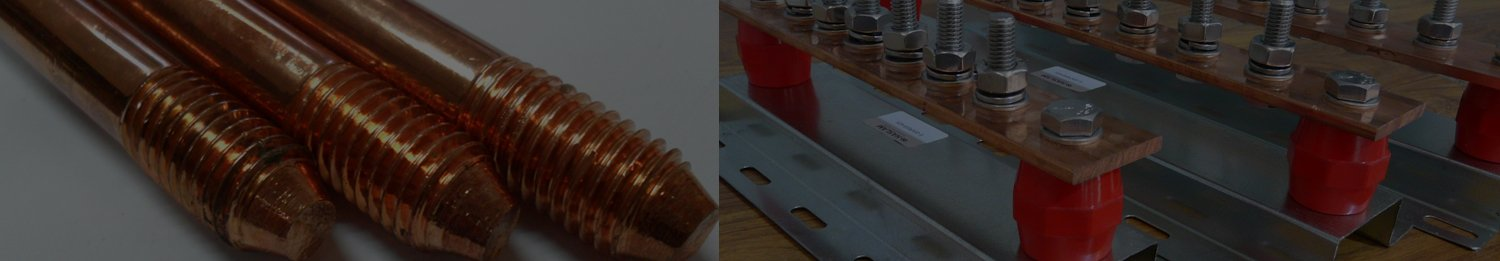# Copper Flat Weight Calculator

Results

To calculate the weight of a copper flat bar, you can use the formula:

Weight = Length × Width × Thickness × Density

Where:

• Length: The length of the flat bar in meters (or any unit you prefer).
• Width: The width of the flat bar in meters (or any unit you prefer).
• Thickness: The thickness of the flat bar in meters (or any unit you prefer).
• Density: The density of copper, which is approximately 8,960 kg/m³ (cubic meters) or 8.96 g/cm³ (grams per cubic centimeter).

Make sure that all the dimensions are in the same unit (e.g., meters or centimeters) before performing the calculation.

Let's work through an example: Suppose you have a copper flat bar with the following dimensions:

• Length: 1 meter
• Width: 5 centimeters
• Thickness: 0.5 centimeters

First, convert all the dimensions to meters:

• Length: 1 meter
• Width: 0.05 meters (5 cm converted to meters)
• Thickness: 0.005 meters (0.5 cm converted to meters)

Density of copper: 8.96 g/cm³ (or 8960 kg/m³)

Now, plug these values into the formula:

Weight = Length × Width × Thickness × Density Weight = 1 m × 0.05 m × 0.005 m × 8960 kg/m³ Weight = 0.224 kg

So, the weight of the copper flat bar is approximately 0.224 kilograms.

Remember that this calculation assumes uniform density and neglects any potential variations due to impurities or manufacturing processes.